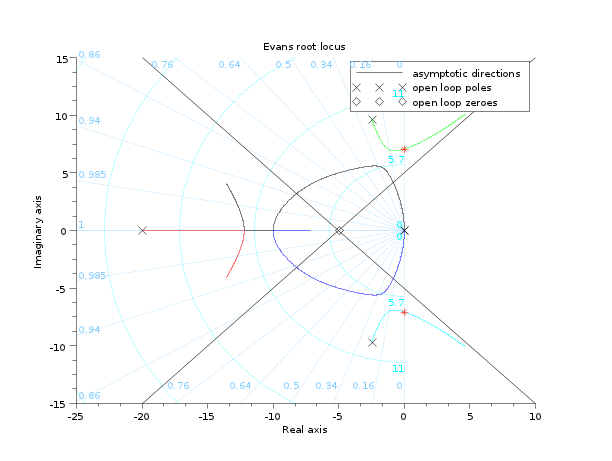Change language to:
Français - 日本語 - Português

See the recommended documentation of this function

Scilab help >> CACSD > evans

# evans

Evans root locus

### Calling Sequence

`evans(H [,kmax])`

### Arguments

H

SISO linear system given by a transfer or a state space representation (see:syslin).

kmax

real (maximum gain desired for the plot )

### Description

Gives the Evans root locus for a SISO linear system in state-space or transfer form H(s) (syslin). This is the locus of the roots of `1+k*H(s)=1+k*N(s)/D(s)`, in the complex plane. For a selected sample of gains ```k <= kmax```, the imaginary part of the roots of `D(s)+k*N(s)` is plotted vs the real part.

To obtain the gain at a given point of the locus you can activate the datatips manager and click the desired point on the root locus.

### Examples

```H=syslin('c',352*poly(-5,'s')/poly([0,0,2000,200,25,1],'s','c'));
clf();evans(H,100);sgrid()
[Ki,s]=kpure(H) // Gains that give pure imaginary closed loop poles
plot([real(s) real(s)],[imag(s) -imag(s)],'*r')

[Kr,s]=krac2(H)```